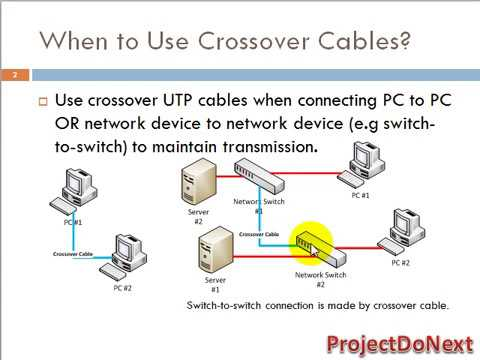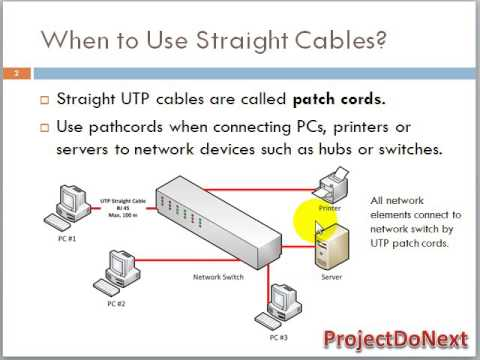# Chapter 1 Subnetting and basics DAY1

CCNA  – Routing & Switching

———–

Physical Address – MAC – 48 Hexa Decimal

IPv4 – it is 32 bit address

xxxxxxxx.xxxxxxxx.xxxxxxx.xxxxxxx

First Octate

Five class

A 0 – 127  –  N.H.H.H (0.0.0.0  = Unkown ip) (127.0.0.0 = loop back ip)

B 128 -191 –  N.N.H.H

C 192 – 223 – N.N.N.H

D 224 – 239 – N.N.N.N  or H.H.H.H  – mutlicast IP

E 240  – 255 Not Used

Note – Unicast = One to One

Multicast = One to Many (a Group)

Subnetmask = it is 32 bit number, used with ipv4 which distinguish network and host part of a given ip.

On bit = network

off bit = host

A = N.H.H.H = 11111111.00000000.00000000.0000000 =  255.0.0.0 /8

B  = N.N.H.H = 1111111.11111111.00000000.0000000 =  255.255.0.0 /16

C  = N.N.N.H = 1111111.11111111.1111111.0000000   = 255.255.0 /24

D =                                                 255.255.255.255 /32

E =

Subnetting = It is breaking of a large network into many small network.

1.FLSM = Fixed length Subnet mask = network is segmented into same size

1. VLSM = Variable lenth subnet mask = network is segmented into difrent size

——————————————————-

192.0.0.0/26

11111111.11111111.1111111.11000000

255.255.255.192

Block Size = 256 – 192 = 64

192.0.0.0  == 192.0.0.1 ——–192.0.0.62    192.0.0.63

192.0.0.64 == 192.0.0.65 ——–192.0.0.126   192.0.0.127

192.0.0.128 == 192.0.0.129——–192.0.0.190   192.0.0.191

192.0.0.192  == 192.0.0.193 ——-192.0.0.254   192.0.0.255

No of n/w = 2^extra on bit = 2^2=4

No of host (ip) = 2^offbit – 2 = 2^6-2 = 64-2 =62

——————————————————

10.0.0.0/24

255.255.255.0

block size of second octat  = 256 – 255  = 1

block size of third octate  = 256-255 =1

no of n/w = 2^16 =??

no of host = 2^8-2 = 256-2=254

10.0.0.0  = 10.0.0.1  —- 10.0.0.254

10.0.1.0   = 10.0.1.1 —– 10.0.1.254

.

.

10.255.255.0 = 10.255.255.1 —– 10.255.255.254

Server LAN = 14     192.168.0.96/28

Staff LAN =  62     192.168.0.0/26

Hr and Admin = 30   192.168.0.64/27

you have been provided class c ip ie 192.168.0.0/24

Rule = Subnet according to larget n/w, then second larget and so on…

No of host (ip) = 2^offbit – 2

Step – 1 for Staff

————–

62              = 2^n-2

2^n=64

n=6  = off bit

32=CIDR+6

CIDR =32-6 =26

192.168.0.0/26 Staff LAN

192.168.0.64/26 Subnet

192.168.0.128/26

192.168.0.192/26

——–

30=2^n-2

32=2^n

n=5

CIDR=32-5= 27

192.168.0.64/27    = Hr and Admin 192.168.0.65 -192.168.0.94

255.255.255.224

Block Size = 256-224 = 32

192.168.0.96/27

Step 3  for Server

—————-

14=2^n-2

2^n=16

n=4

CIDR=32-4= 28

192.168.0.96/28   = server n/w

255.255.255.240

192.168.0.112/28

Types of cable :-